# AP Physics 2 Multiple-select Practice Test 2

### Test Information10 questions18 minutes

1.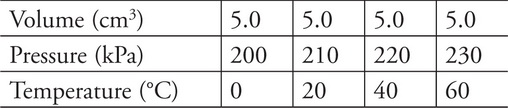A technician is experimenting with a sample of gas in a closed container and produces this set of data. What can be concluded from this data? (Select two answers.)

2. In which of the following cases would it be appropriate to ignore the gravitational force? (Select two answers.)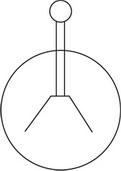Figure 1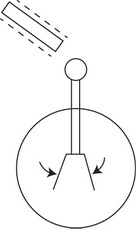Figure 2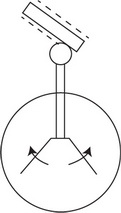Figure 3

3. An electroscope is shown with its movable metal leaves in a sequence of events. Originally the electroscope is in a position with the leaves at an outward angle as shown in Figure 1. A negatively charged rod is brought close to the electroscope and the leaves swing downward as shown in Figure 2. Finally, the rod touches the electroscope and the leaves spring outward as shown in Figure 3. Which of the following statements correctly describe this behavior? (Select two answers.)

4.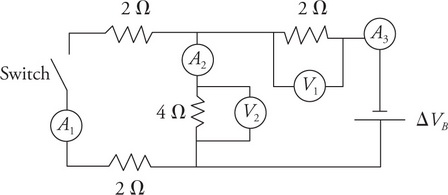The circuit shown has a battery of negligible internal resistance, resistors, and a switch. There are voltmeters, which measure the potential differences V1, and V2, and ammeters A1, A2, A3, which measure the currents I1, I2, and I3. The switch is initially in the closed position. With the switch still closed, which of the following relationships are true? (Select two answers.)

5.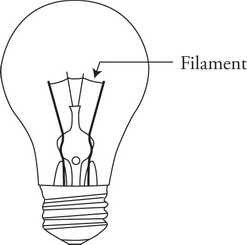An incandescent bulb is shown in the figure. When connected to the same power source, which of the following would make this bulb brighter? (Select two answers.)

6.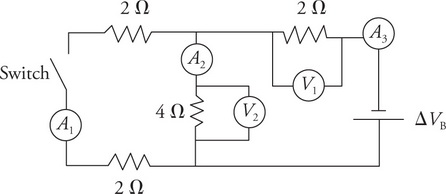The circuit shown has a battery of negligible internal resistance, resistors, and a switch. There are voltmeters, which measure the potential differences V1, and V2, and ammeters A1, A2, A3, which measure the currents I1, I2, and I3. The switch is initially in the closed position. The switch is now opened. Which of the following values increases? (Select two answers.)

7.Particle 1, with a net charge of 1.6 × 10–19C, is injected into a magnetic field directed upward out of the page and follows the path shown in the figure. Particle 2 is then injected into the magnetic field and follows the path shown. Which of the following claims about the particles would be a plausible explanation for the differences in their behavior? (Select two answers.)

8.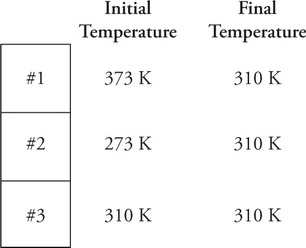Three samples of gas in different containers are put into thermal contact and insulated from the environment as shown in the figure. The three gases, initially at different temperatures, reach a final uniform temperature of 310 K. Which of the following correctly describes the flow of thermal energy from the initial condition until thermal equilibrium? (Select two answers.)

9.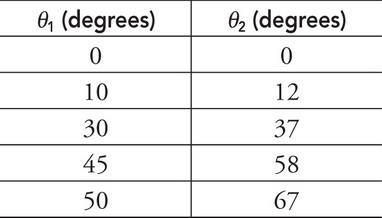In an experiment, students collect data for light traveling from medium 1 into medium 2. The angles of incidence θ1 and refraction θ2 as measured from the perpendicular to the surface are given in the table. The data in the table supports which of the following statements? (Select two answers.)

10. Which of the following phenomena can be better understood by considering the wave-particle duality of electrons? (Select two answers.)